### Time Series Modeling¶

There are several things that are time dependent, I mean, today's values can have an effective relationship to values that have occurred in the past. Some examples related to the subject are demand of products during a certain period, harvest of commodities, stock prices and of course what we will try to predict, the climate change in Rio De Janeiro.

Currently there are several types of time series forecast models, in this notebook I will try to use Seasonal ARIMA Models

First we need to import the essential libraries:

import numpy as np
import pandas as pd
import matplotlib.pyplot as plt
import seaborn as sns
import statsmodels.api as sm
from statsmodels.graphics.tsaplots import plot_acf, plot_pacf
# from sklearn.metrics import mean_squared_error
from math import sqrt
import warnings
warnings.filterwarnings('ignore')
%matplotlib inline

# Reading and transforming the data file
url="https://github.com/jekyll-one/j1-binder-repo/raw/main/data/GlobalLandTemperaturesByMajorCity.csv.zip"
rio = cities.loc[cities['City'] == 'Rio De Janeiro', ['dt','AverageTemperature']]
rio.columns = ['Date','Temp']
rio['Date'] = pd.to_datetime(rio['Date'])
rio.reset_index(drop=True, inplace=True)
rio.set_index('Date', inplace=True)

#I'm going to consider the temperature just from 1900 until the end of 2012
rio = rio.loc['1900':'2013-01-01']
rio = rio.asfreq('M', method='bfill')

Temp
Date
1900-01-31 25.780
1900-02-28 26.160
1900-03-31 23.868
1900-04-30 23.467
1900-05-31 22.667

### The ARIMA models¶

Below I'll try to make a brief explanation about ARIMA models.

##### SARIMA Model (p, d, q)(P, D, Q, S)¶

SARIMA stands for Seasonal Auto Regressive Integrated Moving Average, The name scares, but this is not as scary as it seems.

#### Non seasonal ARIMA¶

We can split the Arima term into three terms, AR, I, MA:

• AR(p) stands for autoregressive model, the p parameter is an integer that confirms how many lagged series are going to be used to forecast periods ahead, example:

• The average temperature of yesterday has a high correlation with the temperature of today, so we will use AR(1) parameter to forecast future temperatures.
• The formula for the AR(p) model is: $\hat{y}_{t} = \mu + \theta_{1}Y_{t-1} + ... + \theta_{p}Y_{t-p}$ Where $\mu$ is the constant term, the p is the periods to be used in the regression and $\theta$ is the parameter fitted to the data.
• I(d) is the differencing part, the d parameter tells how many differencing orders are going to be used, it tries to make the series stationary, example:

• Yesterday I sold 10 items of a product, today I sold 14, the "I" in this case is just the first difference, which is +4, if you are using logarithm base this difference is equivalent to percentual difference.
• If d = 1: $y_{t} = Y_{t} - Y_{t-1}$ where $y_{t}$ is the differenced series and $Y_{t-p}$ is the original series
• If d = 2: $y_{t} = (Y_{t} - Y_{t-1}) - (Y_{t-1} - Y_{t-2}) = Y_{t} - 2Y_{t-1} + Y_{t-2}$
• Note that the second difference is a change-in-change, which is a measure of the local "acceleration" rather than trend.
• MA(q) stands for moving average model, the q is the number of lagged forecast errors terms in the prediction equation, example:
• It's strange, but this MA term takes a percentage of the errors between the predicted value against the real. It assumes that the past errors are going to be similar in future events.
• The formula for the MA(p) model is: $\hat{y}_{t} = \mu - \Theta_{1}e_{t-1} + ... + \Theta_{q}e_{t-q}$ Where $\mu$ is the constant term, q is the period to be used on the $e$ term and $\Theta$ is the parameter fitted to the errors
• The error equation is $e_{t} = Y_{t-1} - \hat{y}_{t-1}$

#### Seasonal ARIMA¶

The p, d, q parameters are capitalized to differ from the non seasonal parameters.

• SAR(P) is the seasonal autoregression of the series.

• The formula for the SAR(P) model is: $\hat{y}_{t} = \mu + \theta_{1}Y_{t-s}$ Where P is quantity of autoregression terms to be added, usually no more than 1 term, s is how many periods ago to be used as base and $\theta$ is the parameter fitted to the data.
• Usually when the subject is weather forecasting, 12 months ago have some information to contribute to the current period.
• Setting P=1 (i.e., SAR(1)) adds a multiple of $Y_{t-s}$ to the forecast for $y_{t}$
• I(D) the seasonal difference MUST be used when you have an strong and stable pattern.

• If d = 0 and D = 1: $y_{t} = Y_{t} - Y_{t-s}$ where $y_{t}$ is the differenced series and $Y_{t-s}$ is the original seasonal lag.
• If d =1 and D = 1: $y_{t} = (Y_{t} - Y_{t-1}) - (Y_{t-s} - Y_{t-s-1}) = Y_{t} - Y_{t-1} -Y_{t-s} + Y_{t-s-1}$
• D should never be more than 1, and d+D should never be more than 2. Also, if d+D =2, the constant term should be suppressed.
• SMA(Q)

• Setting Q=1 (i.e., SMA(1)) adds a multiple of error $e_{t-s}$ to the forecast for $y_{t}$
• S It's the seasonal period where you are going to calculate the the P, D, Q terms. If there is a 52 week seasonal correlation this is the number to be used on the 'S' parameter

#### Trend¶

We will use SARIMAX to create a forecast, the following terms are a definition to the trend:

• 'n' when there is no trend to be used for default.
• ‘c’ indicates a constant, i.e. a degree zero component of the trend polynomial
• ‘t’ indicates a linear trend with time
• ‘ct’ is both trend and constant.
• Can also be specified as an iterable defining the polynomial as in numpy.poly1d

Now, let's plot the series and check how it behaves

plt.figure(figsize=(22,6))
sns.lineplot(x=rio.index, y=rio['Temp'])
plt.title('Temperature Variation in Rio De Janeiro from 1900 until 2012')
plt.show()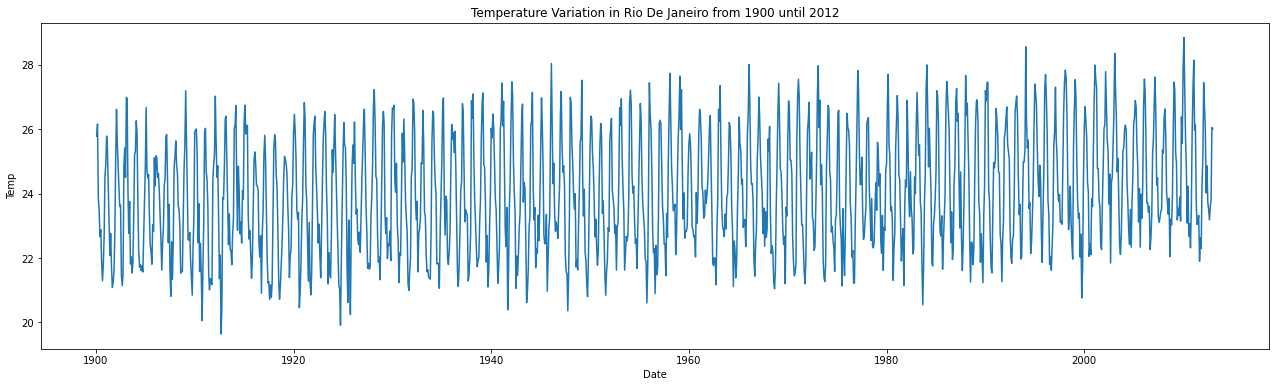# i'm going to create a pivot table to plot the monthly temperatures through the years
rio['month'] = rio.index.month
rio['year'] = rio.index.year
pivot = pd.pivot_table(rio, values='Temp', index='month', columns='year', aggfunc='mean')
pivot.plot(figsize=(20,6))
plt.title('Yearly Rio temperatures')
plt.xlabel('Months')
plt.ylabel('Temperatures')
plt.xticks([x for x in range(1,13)])
plt.legend().remove()
plt.show()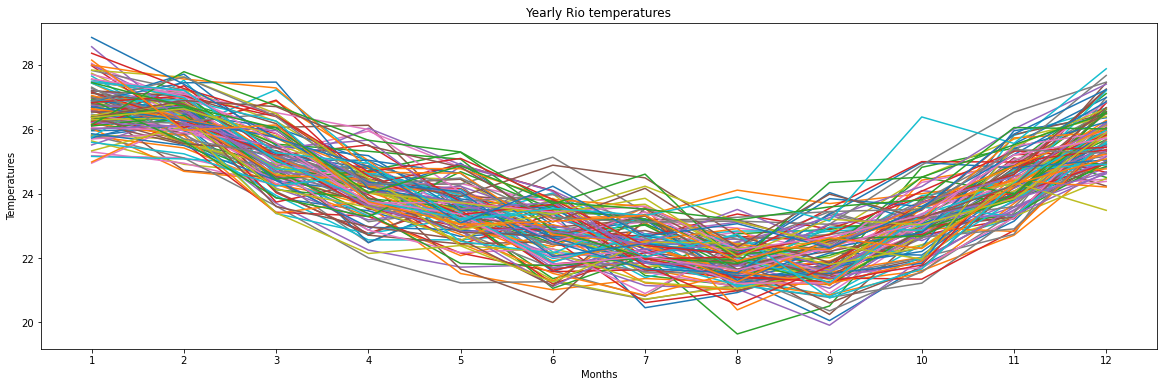The series clearly has some seasonality, the higher temperatures are around November and February and the lower are between July and September. Just to make the things clear, I'll merge these lines into just one line, averaging the monthly levels:

monthly_seasonality = pivot.mean(axis=1)
monthly_seasonality.plot(figsize=(20,6))
plt.title('Monthly Temperatures in Rio De Janeiro')
plt.xlabel('Months')
plt.ylabel('Temperature')
plt.xticks([x for x in range(1,13)])
plt.show()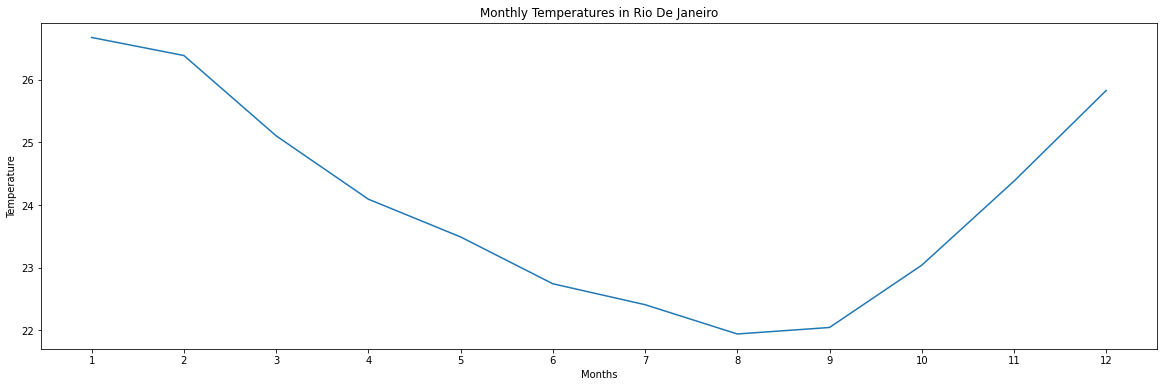Now i'm going to check if there is some trend through the years in this Series:

year_avg = pd.pivot_table(rio, values='Temp', index='year', aggfunc='mean')
year_avg['10 Years MA'] = year_avg['Temp'].rolling(10).mean()
year_avg[['Temp','10 Years MA']].plot(figsize=(20,6))
plt.title('Yearly AVG Temperatures in Rio De Janeiro')
plt.xlabel('Months')
plt.ylabel('Temperature')
plt.xticks([x for x in range(1900,2012,3)])
plt.show()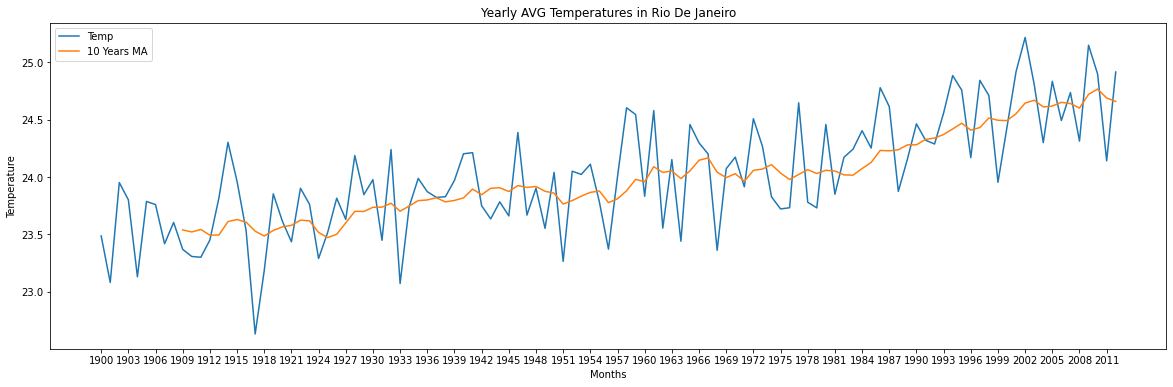We can confirm that there is a constant increasing trend and that the average temperature increased from 23.5º to 24.5º, that's 4.25% in over100 years.

Before we go on, i'm going to split the data in training, validation and test set. After training the model, I will use the last 5 years to do the data validation and test, being 48 months to do a month by month validation and 12 months to make an extrapolation for the future and compare to the test set:

train = rio[:-60].copy()
val = rio[-60:-12].copy()
test = rio[-12:].copy()


And before creating the forecasts we will create a baseline forecast in the validation set, in our simulation we will try to have a smaller error compared to this one.

it will consider the previous month as a base forecast to the next month:

# Excluding the first line, as it has NaN values
baseline = val['Temp'].shift()
baseline.dropna(inplace=True)

Date
2008-02-29    26.362
2008-03-31    26.631
2008-04-30    25.793
2008-05-31    24.031
2008-06-30    23.713
Freq: M, Name: Temp, dtype: float64

Also I'm going to create a function to use the RMSE as a base to calculate the error, but you are free to use another parameter:

def measure_rmse(y_true, y_pred):
#   return sqrt(mean_squared_error(y_true,y_pred))
return np.square(np.subtract(y_true, y_pred)).mean()

# Using the function with the baseline values
rmse_base = measure_rmse(val.iloc[1:,0],baseline)
print(f'The RMSE of the baseline that we will try to diminish is {round(rmse_base,4)} celsius degrees')

The RMSE of the baseline that we will try to diminish is 1.7641 celsius degrees


As we can see, the series has a small uptrend and it appears that there is some seasonality with higher temperatures at the begining and end of the year and lower temperatures around the middle of the year.

To create a time series forecast, the series must be stationary. Constant mean, variance and autocorrelation.

One way to check if the series is stationary is using the adfuller function, if the P-Value is lower than 5%, the series is stationary and you can start creating your model.

If the series isn't stationary you can do some data transformation like using natural logarithm, deflation, differencing, etc.

Below is the function that I used to check the stationarity, it plots:

• The series itself;
• The autocorrelation function (ACF):
• It shows the correlation between the current temperatures versus the lagged versions of itself.
• The partial autocorrelation (PACF):
• It shows the correlation between the current temperatures versus the lagged version excluding the effects of earlier lags, for example, it show the effective influence of the lag 3 in the current temperatures excluding the effects of the lags 1 and 2.

For more interesting sources you can read the materials on this amazing website made by Mr. Robert Nau: Duke University, also you can check Jason Brownlee's website, which have a lot of time series content.

def check_stationarity(y, lags_plots=48, figsize=(22,8)):
"Use Series as parameter"

# Creating plots of the DF
y = pd.Series(y)
fig = plt.figure()

ax1 = plt.subplot2grid((3, 3), (0, 0), colspan=2)
ax2 = plt.subplot2grid((3, 3), (1, 0))
ax3 = plt.subplot2grid((3, 3), (1, 1))
ax4 = plt.subplot2grid((3, 3), (2, 0), colspan=2)

y.plot(ax=ax1, figsize=figsize)
ax1.set_title('Rio De Janeiro Temperature Variation')
plot_acf(y, lags=lags_plots, zero=False, ax=ax2);
plot_pacf(y, lags=lags_plots, zero=False, ax=ax3);
sns.distplot(y, bins=int(sqrt(len(y))), ax=ax4)
ax4.set_title('Distribution Chart')

plt.tight_layout()

print('Results of Dickey-Fuller Test:')

print('\nThe Test Statistics is lower than the Critical Value of 5%.\nThe serie seems to be stationary')
else:
print("\nThe Test Statistics is higher than the Critical Value of 5%.\nThe serie isn't stationary")

# The first approach is to check the series without any transformation
check_stationarity(train['Temp'])

Results of Dickey-Fuller Test:
Test Statistic                   -3.7599
p-value                           0.0033
Lags Used                        23.0000
Number of Observations Used    1272.0000
Critical Value (1%)              -3.4355
Critical Value (5%)              -2.8638
Critical Value (10%)             -2.5680
dtype: float64

The Test Statistics is lower than the Critical Value of 5%.
The serie seems to be stationary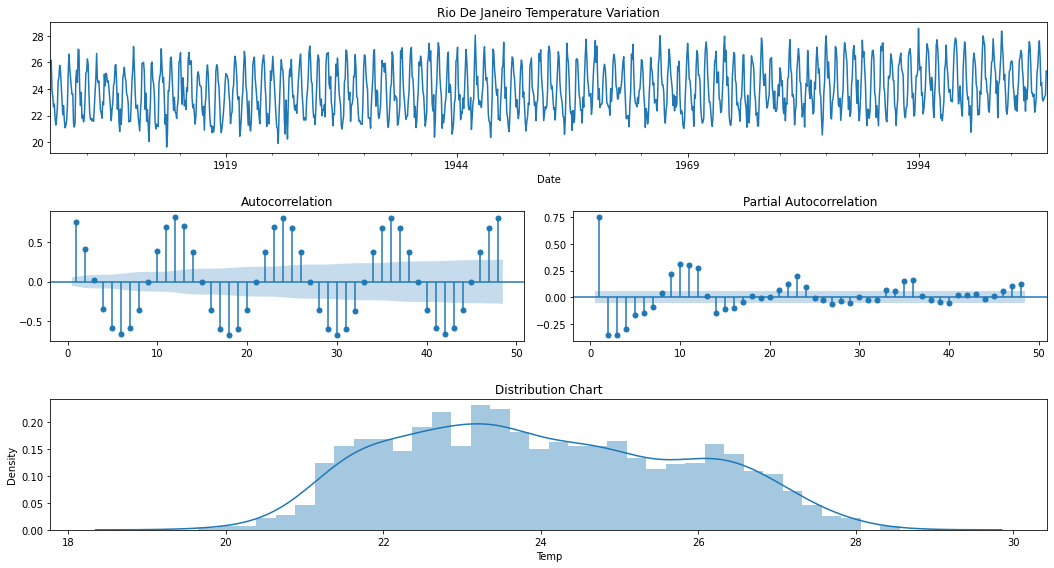The series has an interesting behavior, there is a sequential significative negative autocorrelation starting at lag 6 and repeating each 12 months, it's because of the difference in the seasons, if today is winter with cold temperatures in 6 months we will have higher temperatures in the summer, that's why the negative autocorrelation occurs. These temperatures usually walk in opposite directions.

Also, from lag 12 and sequentially from every 12 lags there is a significant positive autocorrelation. The PACF shows a positive spike in the first lag and a drop to negative PACF in the following lags.

This behavior between the ACF and PACF plots suggests an AR(1) model and also a first seasonal difference ($Y_{t} - Y_{t-12}$). I'll plot the stationarity function again with the first seasonal difference to see if we will need some SAR(P) or SMA(Q) parameter:

check_stationarity(train['Temp'].diff(12).dropna())

Results of Dickey-Fuller Test:
Test Statistic                  -13.4631
p-value                           0.0000
Lags Used                        23.0000
Number of Observations Used    1260.0000
Critical Value (1%)              -3.4356
Critical Value (5%)              -2.8638
Critical Value (10%)             -2.5680
dtype: float64

The Test Statistics is lower than the Critical Value of 5%.
The serie seems to be stationary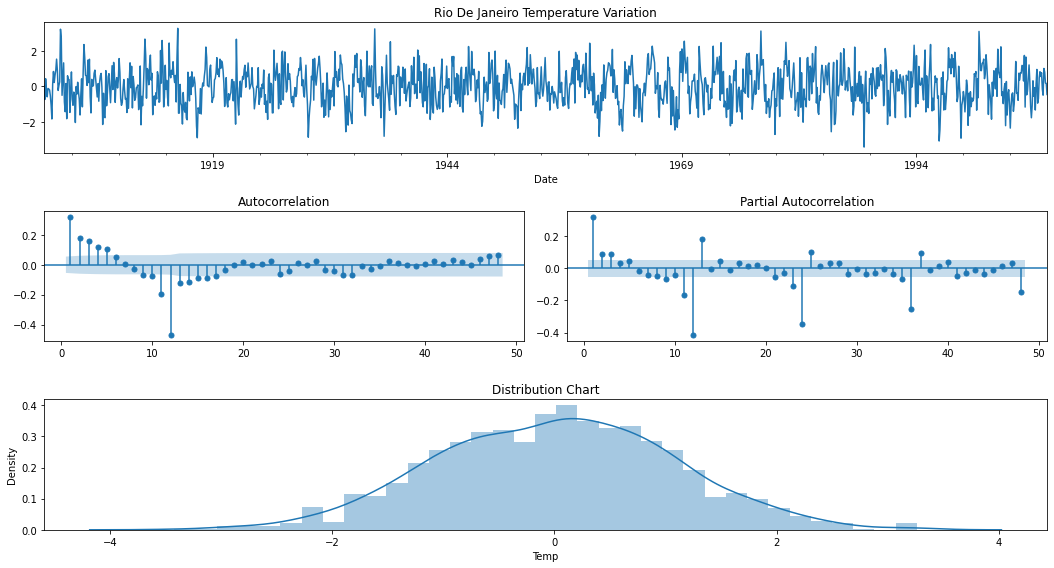As the plots above showed, the first ACF lags have a gradual decay, while the PACF drops under the confidence interval after the third lag, this is an AR signature with a parameter of 3, so this is an AR(3) model.

As we used a first seasonal difference, the ACF and PACF showed a significative drop in the 12th lag, it means an SMA signature with a parameter of 1 lag, resuming this is an SAR(1) with a first difference.

Initially i'm going to work with the following (p,d,q) orders: (3, 0, 0), and with the following seasonal (P, D, Q, S) orders (0,1,1,12) and as the series has a clear uptrend i'm going to use it in the model.

To start forecasting the validation set, I'm going to create a function to use one-step-forecast in the whole validation set and measure the error:

def walk_forward(training_set, validation_set, params):
'''
Params: it's a tuple where you put together the following SARIMA parameters: ((pdq), (PDQS), trend)
'''
history = [x for x in training_set.values]
prediction = list()

# Using the SARIMA parameters and fitting the data
pdq, PDQS, trend = params

#Forecasting one period ahead in the validation set
for week in range(len(validation_set)):
model = sm.tsa.statespace.SARIMAX(history, order=pdq, seasonal_order=PDQS, trend=trend)
result = model.fit(disp=False)
yhat = result.predict(start=len(history), end=len(history))
prediction.append(yhat)
history.append(validation_set[week])

return prediction

# Let's test it in the validation set
train = rio[:-60].copy()
val['Pred'] = walk_forward(train['Temp'], val['Temp'], ((3,0,0),(0,1,1,12),'c'))

# Measuring the error of the prediction
rmse_pred = measure_rmse(val['Temp'], val['Pred'])

print(f"The RMSE of the SARIMA(3,0,0),(0,1,1,12),'c' model was {round(rmse_pred,4)} celsius degrees")
print(f"